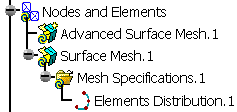# Distributing ElementsThis task shows how to impose nodes (as mesh specifications) either on free edges or on constrained edges.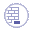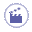1. Click the Element Distribution icon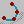from the Local Specifications toolbar.

The Edit Elements Distribution dialog box appears.

• Support: lets you select the edge on each you want to impose nodes.
• Type:
• Uniform: the distance between all the distributed nodes will be the same.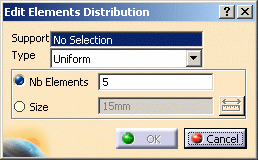• Number of elements: lets you define the element distribution by imposing a number of division on the selected edge.
• Size: lets you define the element distribution by imposing a mesh size on the selected edge.
• Arithmetic: the distance between the distributed nodes will be defined by a common difference computed with the following parameters.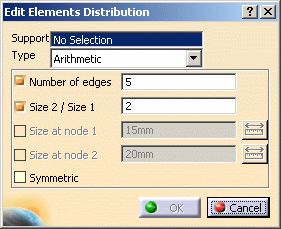• Number of Edges: lets you specify how many edges you want.
• Size 2 / Size 1: lets you specify the ratio between the lengths of the last and the first distribution edge.
• Size at node 1: lets you specify the length of the first edge of the distribution.
• Size at node 2: lets you specify the length of the last edge of the distribution.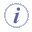Note that you have to specify two and only two parameters among the four.
• Symmetric: lets you specify if the distribution should be symmetric or not.
• Geometric: the distance between the distributed nodes will be defined by a common ratio computed with the following parameters.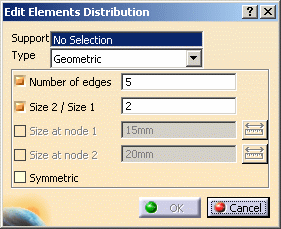• Number of Edges: lets you specify how many edges you want.
• Size 2 / Size 1: lets you specify the ratio between the lengths of the last and the first distribution edge.
• Size at node 1: lets you specify the distance between the two first nodes of the distribution.
• Size at node 2: lets you specify the distance between the two last nodes of the distribution.Note that you have to specify two and only two parameters among the four.
• Symmetric: lets you specify if the distribution should be symmetric or not.
• User Law: the distance between the distributed nodes will be defined by a knowledge law previously defined in the Product Advisor workbench.
For more details about the user law, refer to the Infrastructure User's Guide - Advanced Tasks - Using Knowledgeware Capabilities.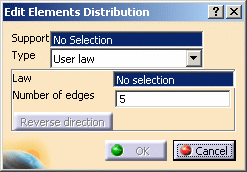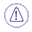A user law must have been defined.
• Law: lets you select the desired law.
• Number of edges: lets specify how many edges you want.
• Reverse direction: this button lets you reverse the direction of distribution.
2. Select the edge on which you want to distribute nodes.

 You can select several edges on the condition they are continuous to each others.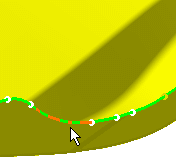3. Set the desired parameters in the Edit Elements Distribution dialog box.

4. If needed, modify the number of the nodes or size. In this case, enter 3 as new value (Number of elements).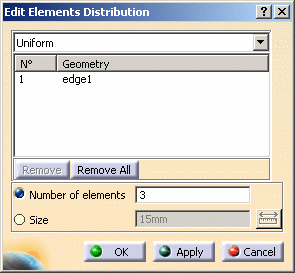5. Click OK in the Edit Elements Distribution dialog box.

 A Mesh Specifications.1 set containing the Elements Distribution.1 specification appears in the specification tree under the Surface Mesh.1 mesh part.